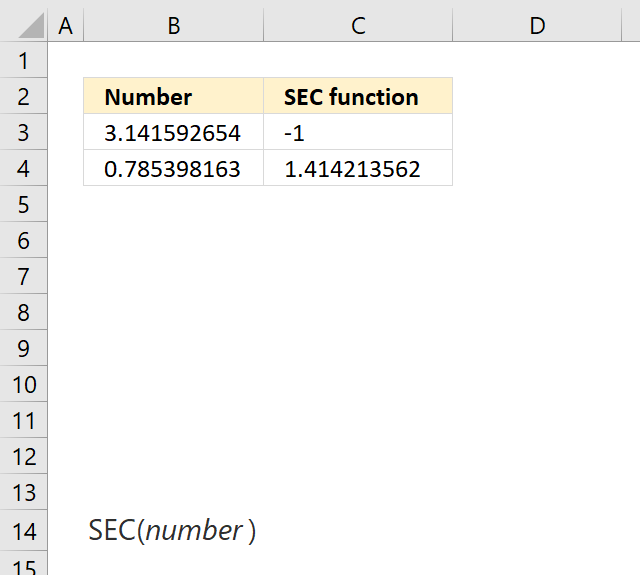Author: Oscar Cronquist Article last updated on May 04, 2018The SEC function calculates the secant of an angle.

Formula in cell E3:

=SEC(B3)

SEC(number)

### Arguments

 number Required. The number (radians) for which you want to calculate the secant.

The secant is calculated like this:

=1/COS(number)